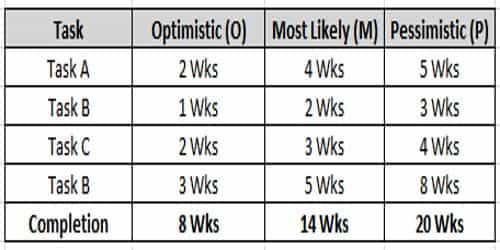# Program Evaluation Review Technique (PERT) AnalysisPERT stands for Program Evaluation Review Technique. It is a method to calculate and estimate the time required to complete a task within deadlines. A PERT chart is a project management tool used to schedule, organize, and coordinate tasks within a project. The major objective of PERT is to decrease the cost and time needed to complete a project.

PERT analysis is a project management planning tool used to estimate the amount of time it will take to practically terminate a project. Steps are involved in PERT analysis:

The following steps are involved in the PERT analysis. These are as follows –

(a) Find out the exertions for project implementation.

(b) Determining the exertion schedule.

(c) Time estimation;

• Optimistic Time;
• Pessimistic Time;
• Most likely Time.

(d) Calculate the estimated Time (TE).

(e) Calculate the variance of the estimated time;

(f) Determine the critical path.

(g) Determine the possibility of a project complete at a specific time.